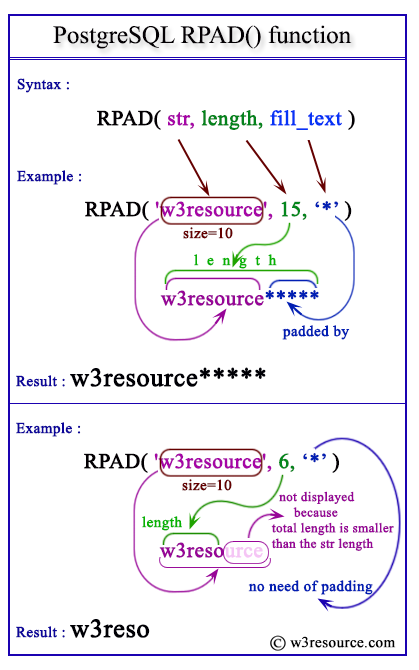The PostgreSQL rpad() function is used to fill up a string of specific length by a substring. If the length of the substring is equal to the remaining length of main string it will fill up properly, if less than the remaining length, the substring will repeat until it is not filling up, if longer than the remaining length or specified length it will be truncated on the left.

Syntax:

`rpad(<string>, length, [<fill_text >])`

Parameters:

Name Description Return Type
string A string, which will be filled up by another string. text
length The length of the string, which will be after filled up by substring.. integer
fill_text The substring which will be fill up the sting to length. text

PostgreSQL Version: 9.3

Pictorial Presentation of PostgreSQL RPAD() functionIn the example below, the 1st parameter within the argument is string i.e. 'w3r', the second one is length, i.e. 10 and the third one is fill_text, i.e. 'esource'. Therefore string has been right padded upto the specific length i.e. 10 by the fill_text i.e. 'esource' and displayed the output.

Code:

``````SELECT rpad('w3r', 10, 'esource');
```
```

Sample Output:

```    rpad
------------
w3resource
(1 row)
```

example 2:

In the example below, the 1st parameter within the argument is string i.e. 'w3r', the second one is length, i.e. 7 and the third one is fill_text, i.e. 'esource'. Therefore string has been right padded upto the specific length i.e. 7 by the fill_text i.e. 'esource' and displayed the output. The point to be noted that the total length of string and fill_text is larger than the length as specified.

Code:

``````SELECT rpad('w3r', 7, 'esource');
```
```

Sample Output:

```  rpad
---------
w3resou
(1 row)
```

Example 3:

In the example below, the 1st parameter within the argument is string i.e. 'w3r', the second one is length, i.e. 14 and the third one is fill_text, i.e. 'esource'. Therefore string has been right padded upto the specific length i.e. 14 by the fill_text i.e. 'esource' and displayed the output. The point to be noted that the total length of string and fill_text is smaller than the length as specified.So, the fill_text right padded the string until the total length is 14

Code:

``````SELECT rpad('w3r', 14, 'esource');
```
```

Sample Output:

```      rpad
----------------
w3resourceesou
(1 row)
```

Previous: REPLACE function
Next: RTRIM function

﻿